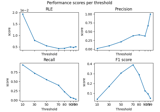# mne.simulation.metrics.region_localization_error#

mne.simulation.metrics.region_localization_error(stc_true, stc_est, src, threshold='90%', per_sample=True)[source]#

Compute region localization error (RLE) between 2 source estimates.

$RLE = \frac{1}{2Q}\sum_{k \in I} \min_{l \in \hat{I}}{||r_k - r_l||} + \frac{1}{2\hat{Q}}\sum_{l \in \hat{I}} \min_{k \in I}{||r_k - r_l||}$

where $$I$$ and $$\hat{I}$$ denote respectively the original and estimated indexes of active sources, $$Q$$ and $$\hat{Q}$$ are the numbers of original and estimated active sources. $$r_k$$ denotes the position of the k-th source dipole in space and $$||\cdot||$$ is an Euclidean norm in $$\mathbb{R}^3$$.

Parameters
stc_trueinstance of (Vol|Mixed)SourceEstimate

The source estimates containing correct values.

stc_estinstance of (Vol|Mixed)SourceEstimate

The source estimates containing estimated values e.g. obtained with a source imaging method.

srcinstance of SourceSpaces

The source space on which the source estimates are defined.

threshold

The threshold to apply to source estimates before computing the dipole localization error. If a string the threshold is a percentage and it should end with the percent character.

per_samplebool

If True the metric is computed for each sample separately. If False, the metric is spatio-temporal.

Returns
metricfloat | array, shape (n_times,)

The metric. float if per_sample is False, else array with the values computed for each time point.

Notes

Papers 1 and 2 use term Dipole Localization Error (DLE) for the same formula. Paper 3 uses term Error Distance (ED) for the same formula. To unify the terminology and to avoid confusion with other cases of using term DLE but for different metric 4, we use term Region Localization Error (RLE).

New in v1.2.

References

1

Kostiantyn Maksymenko, Bernard Giusiano, Nicolas Roehri, Christian-G. Bénar, and Jean-Michel Badier. Strategies for statistical thresholding of source localization maps in magnetoencephalography and estimating source extent. Journal of Neuroscience Methods, 290:95–104, October 2017. doi:10.1016/j.jneumeth.2017.07.015.

2

H. Becker, L. Albera, P. Comon, J. -C. Nunes, R. Gribonval, J. Fleureau, P. Guillotel, and I. Merlet. SISSY: An efficient and automatic algorithm for the analysis of EEG sources based on structured sparsity. NeuroImage, 157:157–172, August 2017. doi:10.1016/j.neuroimage.2017.05.046.

3

Jun Yao and Julius P. A. Dewald. Evaluation of different cortical source localization methods using simulated and experimental EEG data. NeuroImage, 25(2):369–382, April 2005. doi:10.1016/j.neuroimage.2004.11.036.

4

Molins A, Stufflebeam S. M., Brown E. N., and Hämäläinen M. S. Quantification of the benefit from integrating MEG and EEG data in minimum l2-norm estimation. Neuroimage, 42(3):1069–1077, 2008. doi:10.1016/j.neuroimage.2008.05.064.

## Examples using mne.simulation.metrics.region_localization_error#Compare simulated and estimated source activity

Compare simulated and estimated source activity